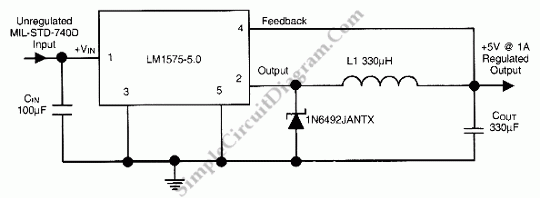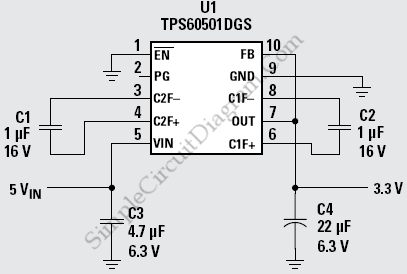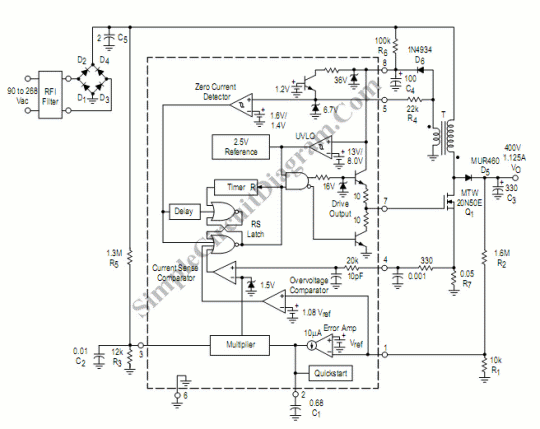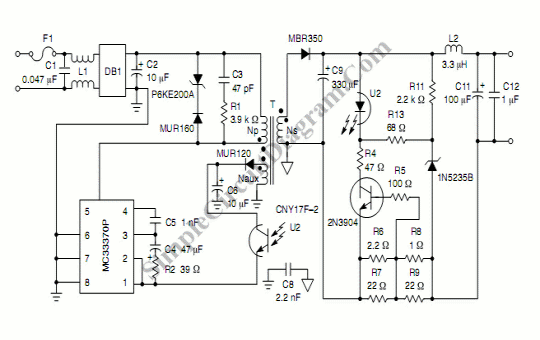## 28V DC To 5V DC Switch-Mode Converter (Regulator)This is a 28VDC to 5VDC switching converter circuit.  As a switch-mode voltage regulator, this circuit gives higher efficiency than linear regulator type. Using the National Semiconductor LM1575-5.0, this circuit can be used to convert 28VDC To 5VDC with efficiency of >80%. This circuit can be used as a 5V source @ 1A. Here is the schematic diagram of the […]

## Step-Down Charge Pump Regulator for USB Powered Devicesthis is a circuit of Step-Down Charge Pump Regulator for USB Powered Devices. The critical design parameter of this circuit is the circuit area. To solve this problem we can use Charge pump step-down circuit. This circuit only requires   the charge pump controller and  four ceramic capacitors. So it is a low cost circuit because of the low cost of […]

## Power Factor ControllerThis circuit is called power factor controller with universal input circuit. It has input range about 90 to 268Vac. Any standards of mains supply  in almost any country would be under that range. Maximum load of this power factor controller is 450W. The circuit shown  by the schematic diagram below : This is a simple circuit because  this circuit uses […]